# Test: Combinational Logic Circuits

## 20 Questions MCQ Test GATE Electrical Engineering (EE) 2023 Mock Test Series | Test: Combinational Logic Circuits

Description
Attempt Test: Combinational Logic Circuits | 20 questions in 60 minutes | Mock test for Railways preparation | Free important questions MCQ to study GATE Electrical Engineering (EE) 2023 Mock Test Series for Railways Exam | Download free PDF with solutions
QUESTION: 1

### A switching function of four variable, f (w, x y, z) is to equal the product of two other function f1 and f2, of the same variable f = f1f2 . The function f and f1 are as follows : f = ∑m(4,7,15) f = ∑m(0,1,2, 3, 4,7, 8,9,10,11,15) Que: The number of full specified function, that will satisfy the given condition, is

Solution:

f = ∑m(4,7,15)

f1 = ∑m(0,1,2, 3, 4,7, 8,9,10,11,15)

f2 = ∑m(4,7,15) + ∑dc(5, 6, 12, 13, 14)

There are 5 don't care condition. So 25 = 32 different functions f2

QUESTION: 2

### A switching function of four variable, f (w, x y, z) is to equal the product of two other function f1 and f2, of the same variable f = f1f2 . The function f and f1 are as follows : f = ∑m(4,7,15) f1 = ∑m(0,1,2, 3, 4,7, 8,9,10,11,15) Que: The simplest function for f2 is

Solution: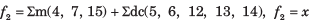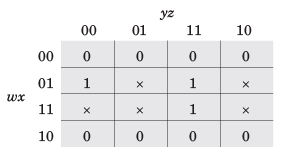QUESTION: 3

### A four-variable switching function has minterms m6 and m9. If the literals in these minterms are complemented, the corresponding minterm numbers are

Solution: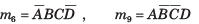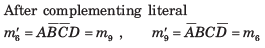QUESTION: 4

The minimum function that can detect a “divisible by 3’’ 8421 BCD code digit (representation D8 D4 D2 D1 ) is given by

Solution:

0, 3, 6 and 9 are divisible by 3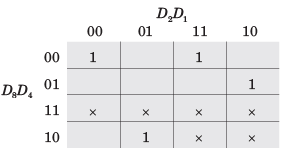f =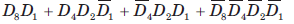QUESTION: 5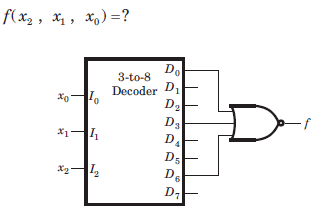Solution: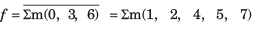QUESTION: 6

For a binary half subtractor having two input A and B, the correct set of logical expressions for the outputs D = (A - B) and X (borrow) are

Solution: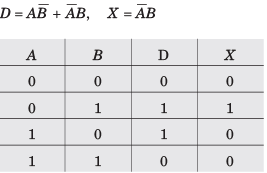QUESTION: 7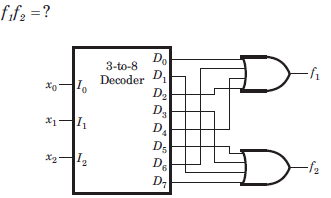Solution: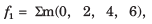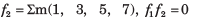QUESTION: 8

What type of logic circuit is represented by the figure shown below?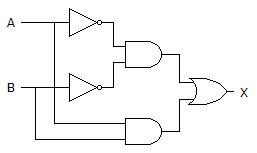Solution:

After solving the circuit we get (A’B’)+AB as output, which is XNOR operation. Thus, it will produce 1 when inputs are even number of 1s or all 0s, and produce 0 when input is odd number of 1s.

QUESTION: 9

The building block shown in fig. is a active high output decoder.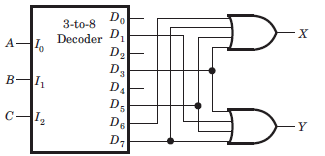Que: The output X is

Solution: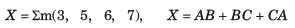QUESTION: 10

The building block shown in fig. is a active high output decoder.Que: The output Y is

Solution: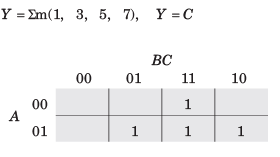QUESTION: 11

A logic circuit consist of two 2 x 4 decoder as shown in fig.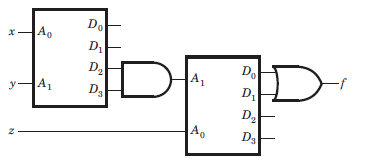The output of decoder are as follow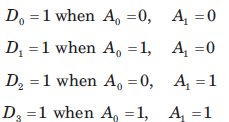The value of f ( x, y, z) is

Solution: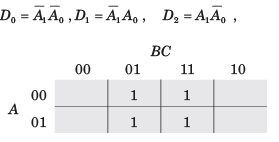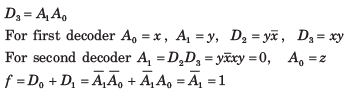QUESTION: 12

A MUX network is shown in fig.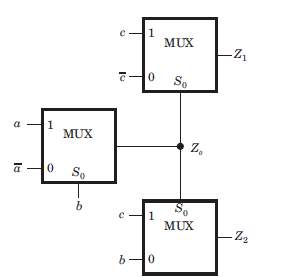Que : Z1 =?

Solution:

The output of first MUX is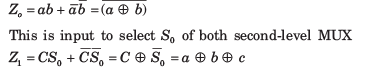QUESTION: 13

How many 3-line-to-8-line decoders are required for a 1-of-32 decoder?

Solution:
QUESTION: 14

A MUX network is shown in fig.Que: This circuit act as a

Solution:

The equation of Z1 is the equation of sum of A and B with carry and equation of 2 is the resultant carry. Thus, it is a full adder.

QUESTION: 15

The network shown in fig. implements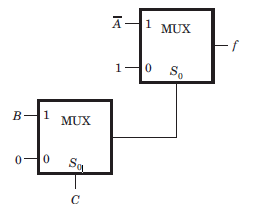Solution:QUESTION: 16

The MUX shown in fig. P4.2.31 is 4 * 1 multiplexer. The output Z is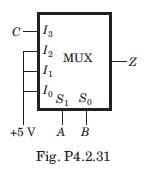Solution:

Explanation : Z = (bar AB)C + (bar A)B + (bar B)A + AB

= (bar A)[(barB)C + B) + A[(bar B) + B]

= (bar A)[(B + C)] + A

= A + B + C

QUESTION: 17

The output of the 4 x 1 multiplexer shown in fig. is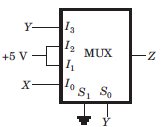Solution:
QUESTION: 18

The MUX shown in fig. is a 4 x 1 multiplexer. The output Z is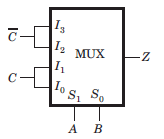Solution: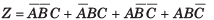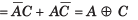QUESTION: 19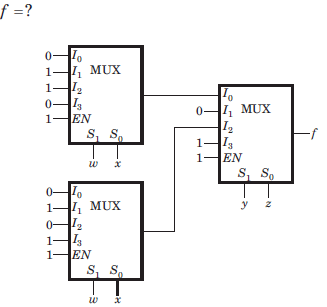Solution:

The output from the upper first level multiplexer is fa and from the lower first level multiplexer is fb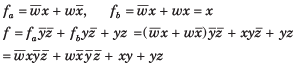QUESTION: 20

For the logic circuit shown in fig.the output Y is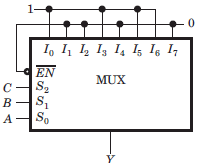Solution: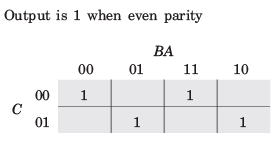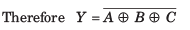Use Code STAYHOME200 and get INR 200 additional OFF Use Coupon Code